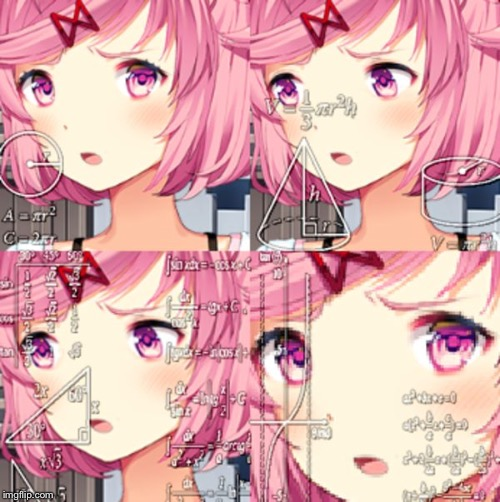# Philosoraptor112 views 5 upvotes Made by Stranger_Things_FAN15 3 years ago in vibingmemesCaption this Meme
1 up, 3y0 ups, 3y
funny meme! have a horse (idk if u like horses take it or leave it)
,
_, ,)\ . ~, , . _
(() ` ` `) \ ) ) ) , , _
| \ ` ` ( ( \ ) ) ) ),, _ ____
| 6 ` | ` ` ( ( \ ( ) ) ) "- ._____.-" ' - . -,
| .'\ ` ` ) ) ) )' \ ) ) )
| | ' ' ' ( ( ( (
\, _) \ | ) ) ) )
` ' \/ ( ( ( ( (
| ) ) ) ) ) )
\ | ( ( ( ( ( (
' | | , ' \ /( ( ( ( ( (
| / ` - . ______ . < \ / ) ) ) ) )
| | / ` . \ | ( ( (

sorry it looks so weird :T
0 ups, 3yCaption this Meme